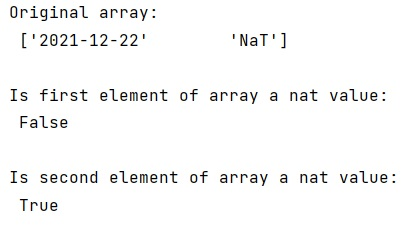# Test array values for NaT (not a time) in NumPy

Learn, how to check whether an array values for NaT (not a time) in NumPy?
Submitted by Pranit Sharma, on January 20, 2023

NumPy is an abbreviated form of Numerical Python. It is used for different types of scientific operations in python. Numpy is a vast library in python which is used for almost every kind of scientific or mathematical operation. It is itself an array which is a collection of various methods and functions for processing the arrays.

## NumPy - Testing array values for NaT (not a time)

Suppose we are given a NumPy array that contains some DateTime values and some NaT values. We need to check if a value is NaT or not.

For this purpose, we can use numpy.isnat() method where we will pass np.datetime64 as a datatype. It will return Boolean values depending on the type of value.

Let us understand with the help of an example,

## Python code to test array values for NaT (not a time) in NumPy

```# Import numpy
import numpy as np

# Creating a numpy array
arr = np.array([np.datetime64('2021-12-22'),np.datetime64('nat')])

# Display original array
print("Original array:\n",arr,"\n")

# Check if a values is nat or not
print("Is first element of array a nat value:\n",np.isnat(arr),"\n")
print("Is second element of array a nat value:\n",np.isnat(arr),"\n")
```

Output:What's New (MCQs)

Top Interview Coding Problems/Challenges!

IncludeHelp's Blogs

Languages: » C » C++ » C++ STL » Java » Data Structure » C#.Net » Android » Kotlin » SQL
Web Technologies: » PHP » Python » JavaScript » CSS » Ajax » Node.js » Web programming/HTML
Solved programs: » C » C++ » DS » Java » C#
Aptitude que. & ans.: » C » C++ » Java » DBMS
Interview que. & ans.: » C » Embedded C » Java » SEO » HR
CS Subjects: » CS Basics » O.S. » Networks » DBMS » Embedded Systems » Cloud Computing
» Machine learning » CS Organizations » Linux » DOS
More: » Articles » Puzzles » News/Updates